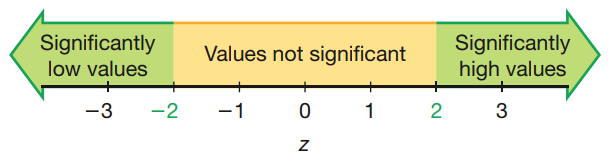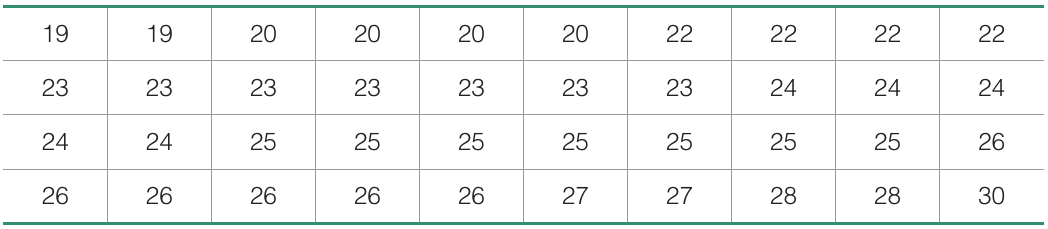# Measures of relative standing

02/13/2020

## Instructions:

Use the left and right arrow keys to navigate the presentation forward and backward respectively. You can also use the arrows at the bottom right of the screen to navigate with a mouse.

FAIR USE ACT DISCLAIMER:
This site is for educational purposes only. This website may contain copyrighted material, the use of which has not been specifically authorized by the copyright holders. The material is made available on this website as a way to advance teaching, and copyright-protected materials are used to the extent necessary to make this class function in a distance learning environment. The Fair Use Copyright Disclaimer is under section 107 of the Copyright Act of 1976, allowance is made for “fair use” for purposes such as criticism, comment, news reporting, teaching, scholarship, education and research.

## Outline

• The following topics will be covered in this lecture:
• Z scores
• Percentiles
• Quartiles
• Box plots

## Z scores

• We will start to look at measures of relative standing.

• Measures of relative standing are tools to describe the location of observations in a data set with respect to the other data pieces.
• The most important measure of relative standing is the z score;

• a z score utilizes our understanding of the spread and concentration of normal data in terms of the standard deviation.
• Like the coefficient of variation, we will make this score into a measure on a relative scale so we can compare values from different distributions.

• Specifically, suppose we have a sample value $$x$$ from a normal data set with sample mean $$\overline{x}$$ and sample standard deviation $$s$$.

• Suppose that the population mean is $$\mu$$ and the population standard deviation is $$\sigma$$.

• The z score of $$x$$ is given as

$\begin{matrix} \text{Sample z score} = \frac{x - \overline{x}}{s} & & \text{Population z score} = \frac{x - \mu}{\sigma} \end{matrix}$

• This measures how far $$x$$ deviates from the mean, relative to the size of standard deviation.

• Note, we will typically round the z score to two decimal places.

• Z scores also apply to non-normal data, but their interpretation changes slightly as we cannot use the empirical rule in this context.

## Interpreting z scoresCourtesy of Mario Triola, Essentials of Statistics, 6th edition

• Let us recall the empirical rule for normally distributed data:
• Approximately $$68\%$$ of the sample data will lie within one standard deviation $$\sigma$$ of the population mean $$\mu$$, i.e., in $[\mu - \sigma, \mu + \sigma].$
• Approximately $$95\%$$ of sample data will lie within two standard deviations $$2\sigma$$ of the population mean $$\mu$$, i.e., in $[\mu - 2\sigma, \mu + 2\sigma].$
• Approximately $$99.7\%$$ of sample data will lie within three standard deviations $$3\sigma$$ of the population mean $$\mu$$, i.e., in $[\mu - 3\sigma, \mu + 3\sigma].$
• By convention, we will say that an observation is statistically significant low or high in value if there is $$5\%$$ or less chance to observe a value at least as extreme.
• Discuss with a neighbor: if an observation from a normal data set has a z score of $$1$$ is this significant? Why? What is the probability of finding a value at least this extreme?
• By the empirical rule, there is a $$68\%$$ chance of finding a value within one standard deviation.
• Therefore, $$100\% - 68\% = 32\%$$ of values lie outside of one standard deviation – i.e., they are at least this extreme. This is not significant.
• Discuss with a neighbor: if an observation from a normal data set has a z score of $$2$$ is this significant? Why? What is the probability of finding such a value?
• By the empirical rule, there is a $$95\%$$ chance of finding a value within two standard deviations, so $$100\% - 95\% = 5\%$$ of values lie outside of two standard deviations – i.e., they are at least this extreme. This is significant.

### Interpreting z scores continuedCourtesy of Mario Triola, Essentials of Statistics, 6th edition

• Less formally, if the data is not normally distributed we will still use the range rule of thumb as an approximation of the empirical rule.
• If the data is not normally distributed, the empirical rule no longer applies, i.e.,
• We are not guaranteed that $$68\%$$ of data will lie within one standard deviation of the mean.
• We are not guaranteed that $$95\%$$ of data will lie within two standard deviations of the mean.
• We are not guaranteed that $$99.7\%$$ of data will lie within three standard deviations of the mean.
• However, Chebyshev’s theorem says that at least $$75\%$$ of data lies within two standard deviations of the mean.
• The actual amount will often be more than this, as this is a lower bound on any data set.
• Therefore, we can still consider a z score of 2 to be interesting for non-normal data, but we must be more careful about our conclusions.

### Interpreting z scores example

• Discuss with a neighbor: which of the following two values is more extreme from the data set from which it came?
1. A baby is born with weight $$4000.0g$$, where the sample data includes $$400$$ babies with sample mean $$\overline{x}=3152.0g$$ and sample standard deviation $$s=693.4g$$
2. An adult is measured with a body temperature of $$99^\circ F$$ out of sample data of $$106$$ adults with sample mean $$\overline{x}=98.20^\circ F$$ and sample standard deviation of $$0.62^\circ F$$.
• To compare these two measurements which exist on different scales and units, we compute their z scores as: $\begin{matrix} \text{baby z score}=\frac{4000.0g - 3152.0g}{693.4g} = 1.22\text{ std} & & \text{heat z score}=\frac{ 99^\circ F - 98.2^\circ F}{0.62^\circ F} = 1.29\text{ std} \end{matrix}$
• By comparing the z scores, we see that the body temperature measurment is more standard deviations away from its sample mean than the baby’s weight.
• Even though the difference in temperature units is small, the relatively small standard deviation in the measurements makes this a more extreme value with respect to its sample data set.
• This illustrates the purpose of the z score, in that it makes all measurements comparable on a relative, standardized scale.
• We note that the z score is signed with a $$\pm$$. One important property of the z score is that it tells whether the value lies above or below the mean.
• In the above both measurements lie above the mean value of the samples and for this reason they are positive;
• on the other hand, whenever we see a negative z score, we know already that the measurement was below the mean of the samples.

## Percentiles

• Percentiles – these are measures of location, denoted $$P_1, P_2,\cdots, P_{99}$$, which divide a set of data into $$100$$ groups with about $$1\%$$ of the values in each group.

• An example we know already is the median.
• Indeed, the median is the $$P_{50}$$ percentile, which separates the data into groups with $$50\%$$ of the data above and $$50\%$$ of the data below.
• There are different ways in which the percentile can be computed, and therefore we will consider one of several possible approaches;

• the important part is to understand how we can convert a data value into a percentile, and
• how to convert a percentile back into a data value.
• We will discuss both of these in the following, but note,

• converting back and forth, the results can be inconsistent.
• We should be careful therefore about what is the question at hand.

### Converting data into percentiles

• Suppose we have samples given as $$x_1, \cdots, x_n$$ where $$n$$ is the total number of samples in the data set.

• Suppose the measurements are quantitative, so that we can arrange the samples in order;

• that is, up to re-naming samples, we can write $x_i \leq x_{i+1}$ for each $$i = 1,\cdots, n-1$$.
• Then, for a particular value $$x$$, its percentile can be computed as,

\begin{align}\text{Percentile of }x &= \frac{\text{Number of samples with value less than } x}{\text{Number of total samples}}\times 100 \end{align}

• If we can order the sample values as above, we thus look for the index $$i$$ for which $x_i < x \leq x_{i+1}$
• That is, we count the number of samples $$i$$ with value strictly less than $$x$$;
• the next ordered sample $$x_{i+1}$$ can have a value that is either greater than or equal to the value $$x$$.
• If we choose the index $$i$$ as above, the formula becomes \begin{align}\text{Percentile of }x &= \frac{i}{n}\times 100 \end{align}

### Finding the percentile of some valueCourtesy of Mario Triola, Essentials of Statistics, 5th edition

• In the table to the left, we see an example data set where the samples have been ordered from low to high in the value.
• There are $$4$$ rows and $$10$$ columns to this table.
• The samples are the number of chocolate chips in a batch of 40 cookies.
• Discuss with a neighbor: what is the percentile of $$x=23$$? That is, what is the percent of samples that have value lower than $$23$$, relative to the total number of samples?
• Notice there are $$10$$ columns and the first row consists of samples with value less than $$23$$.
• That is to say, $x_{10} < 23 \leq x_{11}.$
• In this regard, we have, $\text{Percentile of }23 = \frac{10}{40}\times 100 = 25.$
• Therefore, we say $$x=23$$ is in the $$25$$-th percentile.
• Similar to the median, we can say that a cookie with $$23$$ chips approximately separates the cookies with the lowest $$25\%$$ of chips from those with the highest $$75\%$$ of chips.
• Note: we do not say $$P_{25}=23$$, we will show how to find $$P_{25}$$ in the following.